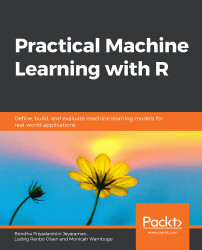•#### Practical Machine Learning with R#### Overview of this book

With huge amounts of data being generated every moment, businesses need applications that apply complex mathematical calculations to data repeatedly and at speed. With machine learning techniques and R, you can easily develop these kinds of applications in an efficient way. Practical Machine Learning with R begins by helping you grasp the basics of machine learning methods, while also highlighting how and why they work. You will understand how to get these algorithms to work in practice, rather than focusing on mathematical derivations. As you progress from one chapter to another, you will gain hands-on experience of building a machine learning solution in R. Next, using R packages such as rpart, random forest, and multiple imputation by chained equations (MICE), you will learn to implement algorithms including neural net classifier, decision trees, and linear and non-linear regression. As you progress through the book, you’ll delve into various machine learning techniques for both supervised and unsupervised learning approaches. In addition to this, you’ll gain insights into partitioning the datasets and mechanisms to evaluate the results from each model and be able to compare them. By the end of this book, you will have gained expertise in solving your business problems, starting by forming a good problem statement, selecting the most appropriate model to solve your problem, and then ensuring that you do not overtrain it.Free Chapter
An Introduction to Machine LearningData Cleaning and Pre-processingFeature EngineeringIntroduction to neuralnet and Evaluation MethodsLinear and Logistic Regression ModelsUnsupervised LearningAppendix## Summary

In this chapter, you trained, evaluated, and compared multiple neural network architectures on the GermanCredit and PimaIndiansDiabetes2 classification tasks. To achieve this, you created balanced partitions and folds with the groupdata2 package. You used the neuralnet package to specify and train neural networks and used those trained models to predict the classes in the development and validation sets. Both in theory and by using caret's confusionMatrix function, you learned how to calculate accuracy, precision, recall, and F1 metrics. You implemented a cross-validation training loop and used it to compare multiple model architectures. Finally, we introduced multiclass classification and the softmax function.

If you wish to build more advanced neural networks while keeping the code simple, the keras package would be a good place to start.

In the next chapter, you will learn how to fit and interpret linear and logistic regression models. We will use the cvms package to easily...